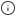# Counter aggregates

When you process counter data, it is usually assumed that if the value of the counter goes down, the counter has been reset. For example, if you wanted to count the total number of miles travelled in a vehicle, you would expect the values to continuously increase: 1, 2, 3, 4, and so on. If the counter reset to 0, you would expect that this was a new trip, or an entirely new vehicle. This can become a problem if you want to continue counting from where you left off, rather than resetting to 0. A reset could occur if you have had a short server outage, or any number of other reasons. To get around this, you can analyze counter data by looking at the change over time, which accounts for resets.

Accounting for resets can be difficult to do in SQL, so Timescale has developed aggregate and accessor functions that handle calculations for counters in a more practical way.###### Note

Counter aggregates can be used in continuous aggregates, even though they are not parallelizable in PostgreSQL. For more information, see the section on parallelism and ordering.

## Run a counter aggregate query using a delta function

In this procedure, we are using an example table called `example` that contains counter data.

### Running a counter aggregate query using a delta function

1. Create a table called `example`:

`CREATE TABLE example (    measure_id      BIGINT,    ts              TIMESTAMPTZ ,    val             DOUBLE PRECISION,    PRIMARY KEY (measure_id, ts));`
2. Create a counter aggregate and the delta accessor function. This gives you the change in the counter's value over the time period, accounting for any resets. This allows you to search for fifteen minute periods where the counter increased by a larger or smaller amount:

`SELECT measure_id,    delta(        counter_agg(ts, val)    )FROM exampleGROUP BY measure_id;`
3. You can also use the `time_bucket` function to produce a series of deltas over fifteen minute increments:

`SELECT measure_id,    time_bucket('15 min'::interval, ts) as bucket,    delta(        counter_agg(ts, val)    )FROM exampleGROUP BY measure_id, time_bucket('15 min'::interval, ts);`

## Run a counter aggregate query using an extrapolated delta function

If your series is less regular, the deltas are affected by the number of samples in each fifteen minute period. You can improve this by using the `extrapolated_delta` function. To do this, you need to provide bounds that define where to extrapolate to. In this example, we use the `time_bucket_range` function, which works in the same way as `time_bucket` but produces an open ended range of all the times in the bucket. This example also uses a CTE to do the counter aggregation, which makes it a little easier to understand what's going on in each part.

### Running a counter aggregate query using an extrapolated delta function

1. Create a table called `example`:

`CREATE TABLE example (    measure_id      BIGINT,    ts              TIMESTAMPTZ ,    val             DOUBLE PRECISION,    PRIMARY KEY (measure_id, ts));`
2. Create a counter aggregate and the extrapolated delta function:

`with t as (    SELECT measure_id,        time_bucket('15 min'::interval, ts) as bucket,        counter_agg(ts, val, time_bucket_range('15 min'::interval, ts))    FROM example    GROUP BY measure_id, time_bucket('15 min'::interval, ts))SELECT time_bucket,    extrapolated_delta(counter_agg, method => 'prometheus')FROM t ;`###### Note

In this procedure, `Prometheus` is used to do the extrapolation. Timescale's current `extrapolation` function is built to mimic the Prometheus project's `increase` function, which measures the change of a counter extrapolated to the edges of the queried region.

## Run a counter aggregate query with a continuous aggregate

Your counter aggregate might be more useful if you make a continuous aggregate out of it.

### Running a counter aggregate query with a continuous aggregate

1. Create a hypertable partitioned on the `ts` column:

`SELECT create_hypertable('example', 'ts', chunk_time_interval=> '15 days'::interval, migrate_data => true);`
2. Create the continuous aggregate:

`CREATE MATERIALIZED VIEW example_15WITH (timescaledb.continuous)AS SELECT measure_id,    time_bucket('15 min'::interval, ts) as bucket,    counter_agg(ts, val, time_bucket_range('15 min'::interval, ts))FROM exampleGROUP BY measure_id, time_bucket('15 min'::interval, ts);`
3. You can also re-aggregate from the continuous aggregate into a larger bucket size:

`SELECT    measure_id,    time_bucket('1 day'::interval, bucket),    delta(        rollup(counter_agg)    )FROM example_15GROUP BY measure_id, time_bucket('1 day'::interval, bucket);`

## Parallelism and ordering

The counter reset calculations require a strict ordering of inputs, which means they are not parallelizable in PostgreSQL. This is because PostgreSQL handles parallelism by issuing rows randomly to workers. However, if your parallelism can guarantee sets of rows that are disjointed in time, the algorithm can be parallelized, as long as it is within a time range, and all rows go to the same worker. This is the case for both continuous aggregates and for distributed hypertables, as long as the partitioning keys are in the `group by`, even though the aggregate itself doesn't really make sense otherwise.